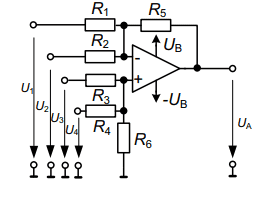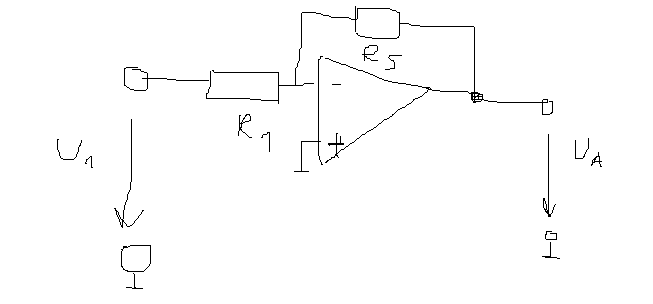# Why is the output voltage of the subtractor calculated like this?

• Engineering
• arhzz
It is not the first time, but I have 0 experience with OP amps. We just learned it in this class and this is the first homework regarding OP amps so I assumed that I was at fault.

#### arhzz

Homework Statement
Find the output voltage
Relevant Equations
Circuit analysis
Hello! Consider this operational amplifier circuit,a subtractor to be more preciseI need to find the Ua (output voltage) using superposition; Now I dont have any values given for the resistors but that they are all the same (R1=R2=R3=R4=R5) so I just need to find the expressions.I've tried it like this. I let U1 be active and all of the other voltages get short circuited. When I simplify the circuit in that way I get a simple inverting operational amplifier that looks like this;By no means a pretty picture but I think it will suffice. So I get that Ua is simply ## U_{A} = -\frac{R5}{R1}U_1## And according to the solutions this is correct. Now I did the same thing for U2 and I get the same circuit (simplified) but the first resistor is R2 and not R2 so the voltage is ##U_{A} = -\frac{R5}{R2}U_2 ## But according to the solutions it shouldnt be the resistor R2 but rather the resistor R1

It states "Same circuit as for U1" and gives the same equation but with voltage U2. I do not understand this; how can it be the same resistor? If we put it on ground and give voltage to R2 how can we get the same circuit if we do it vice versa? I'd assume I am simplifying the circuit wrong,that is why I posted the second picture,is the simplified circuit correct?

I don't understand your derivation, having all other voltages shorted out doesn't mean all other resistors related to those voltages disappear accordingly.

The resistances in series with the shorted voltage sources will have 0V on both the terminals. So the simplified circuit looks correct to me.

cnh1995 said:
The resistances in series with the shorted voltage sources will have 0V on both the terminals. So the simplified circuit looks correct to me.
Yea that was my idea as well.

arhzz said:
Now I did the same thing for U2 and I get the same circuit (simplified) but the first resistor is R2 and not R2 so the voltage is ##U_{A} = -\frac{R5}{R2}U_2 ## But according to the solutions it shouldnt be the resistor R2 but rather the resistor R1
Is this the first time for you to find a wrong statement in a solution manual? You never should blindly follow any printed statements.

LvW said:
Is this the first time for you to find a wrong statement in a solution manual? You never should blindly follow any printed statements.
It is not the first time, but I have 0 experience with OP amps. We just learned it in this class and this is the first homework regarding OP amps so I assumed that I was at fault.